ISSN: 1070-4698

# Vol. 44

Latest Volume
All Volumes
All Issues

PIER Vol. 44, 309-333, 2004. doi:10.2528/PIER03073001

###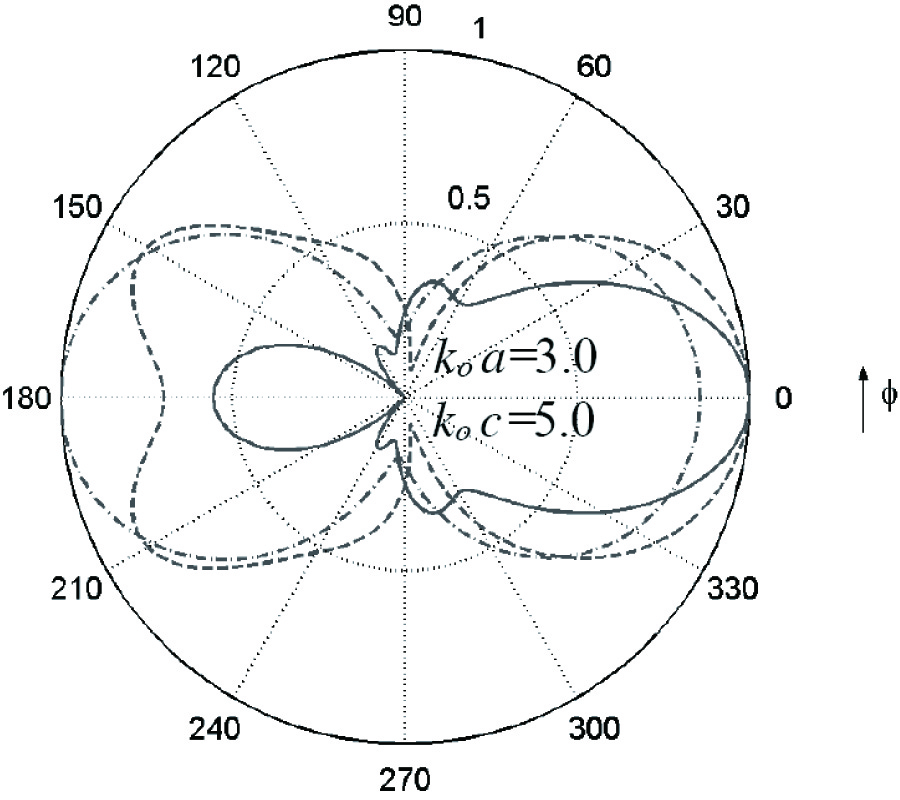Radiation Characteristics of an Axially Asymmetrical Slot Antenna on a Perfectly-Conducting Prolate Spheroid Coated with Homogeneous Materials

Ming Zhang and Abdel Sebak

The boundary value solution of electromagnetic radiation from an axis-asymmetric slot antenna on a perfectly conducting prolate spheroid coated with a confocal sheath is presented. The electromagnetic fields are expanded in terms of prolate spheroidal vector wave functions. The unknown expansion coefficients are determined from a set of linear equations derived from the application of boundary conditions on the tangential fields' components. Numerical results for radiation patterns and power are presented. It is found that the thickness of the sheath has a significant effect on the radiated fields, and the radiated power is greatly enhanced for certain values of the sheath thickness.

PIER Vol. 44, 287-308, 2004. doi:10.2528/PIER03080801

###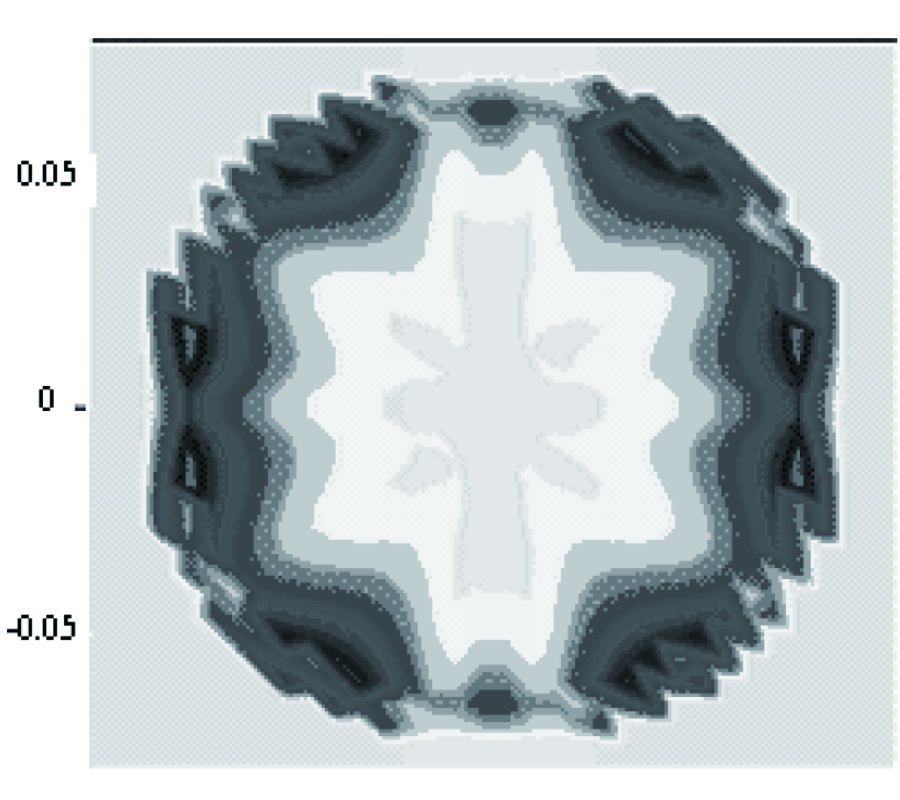Electromagnetic Analysis of a Non-Invasive 3D Passive Microwave Imaging System

Irene Karanasiou, Nikolaos Uzunoglu, and Anastasios Garetsos

A technique based on the Green's function theory is used in the present research in order to study theoretically the focusing properties of a constructed 3D non-invasive microwave imaging system, consisting of an ellipsoidal conductive cavity and a radiometric receiver. A double layered spherical human head model is placed on one focal point of the elliptical reflector, while the receiving antenna is placed on the other focus. Making use of the reciprocity theorem, the equivalent problem of the coupling between an elementary dipole and the double layered lossy dielectric human spherical model is solved. Numerical results concerning the electric field distribution inside the head model and in the rest of the cavity, at two operating frequencies (1.5 GHz and 3.5 GHz), are presented and compared to the results of an electromagnetic simulator. Finally, phantom experimental results validate the proof of concept and determine the temperature and spatial attributes of the system.

PIER Vol. 44, 267-286, 2004. doi:10.2528/PIER03061101

### Impedance Boundary Conditions on a Chiral Film

Pierre Hillion

Using a technique borrowed from Idemen  and requiring the Fourier transform of the x, y-components of the electric and magnetic fields, we obtain the impedance boundary conditions for electromagnetic plane waves withh orizontal, vertical and arbitrary polarization incident on a infinite, smooth, chiral film located at z = 0 and deposited on a metallic substrate. As an application, we discuss the scattering of harmonic plane waves and of a finite beam on sucha film.

PIER Vol. 44, 249-266, 2004. doi:10.2528/PIER03060501

###The Surface Impedance of a Homogeneous TM-Type Plane Wave at Skew Incidence Upon an Inclined Anisotropic Half-Space

Glenn Wilson and David V. Thiel

General expressions for the electromagnetic fields of homogeneous TM-type plane waves at a skew angle of incidence upon an inclined anisotropic half-space are derived. Previous analyses have only considered fields of homogeneous plane waves in the problems of a laterally anisotropic half-space, and not the problem of an inclined anisotropic half-space. Previous analyses also have assumed that the linear polarization of the incident magnetic field is maintained, regardless of the anisotropy present. The results presented in this paper have shown that while this assumption is valid only for the magnetic field, the electric field is elliptically polarised in the anisotropic half-space. This is demonstrated through a model study and experimental verification at VLF. The solutions obtained converge on the expected values for the special cases presented by other authors.

PIER Vol. 44, 231-248, 2004. doi:10.2528/PIER03060301

###Wide-Angle Radar Target Recognition with Subclass Concept

Dong-Kyu Seo, Kyung-Tae Kim, In-Sik Choi, and Hyo-Tae Kim

The range profile is an easily obtainable and promising feature vector for a real-time radar target recognition system. However, the range profile is highly dependent on the aspect angle of a target. This dependency makes the recognition over a wide angular region difficult. In this paper, we propose a classifier with a subclass concept in order to solve this dependency problem. Recognition results with six aircraft models measured at a compact range facility are presented to show the effectiveness of the proposed classifier over a wide-angular region.

PIER Vol. 44, 217-229, 2004. doi:10.2528/PIER03052001

###Cooperative Targets Detection and Tracking Range Maximization Using Multimode Ladar/Radar and Transponders

A LAser raDAR (LADAR) system was described in previous papers for detecting and tracking cooperative targets. The LADAR system was optimized to achieve accurate tracking with a high probability of detection and low False Alarm Rate (FAR). However, the operation range was limited to about thirty km's under clear sky conditions and less in low visibility and bad weather conditions. To obtain operation ranges in the order of hundreds km's without affecting tracking accuracy a LADAR/RADAR dual mode system was developed. Moreover, very bulky, expensive and powerful RADAR equipments are required. In this paper, we propose a tactical mobile tracking LADAR/RADAR systems based on a multi-mode LADAR/RADAR combination using active transponders on cooperative targets for predetection and tracking at higher range distances. The optimal solution described in this paper is based on a six-step multimode operation procedure, which starts from an L-band active transponder for maximal distance detection and tracking, and with decreasing distance it switches to an L-band RADAR , Ka-band RADAR with and without active transponder, LADAR with transponder and then to a LADAR without transponder for the final tracking step. Although relatively complicated (6 steps), our proposed solution requires significantly lower power levels and produces less radio interference than the former dualmode system as shown in Table 1.

PIER Vol. 44, 197-215, 2004. doi:10.2528/PIER03051201

###The Proper Current Spectra of an Open Integrated Microstrip Waveguide

Continuous current spectrum of an integrated open waveguide structure is identified as the branch cut contribution to singularity expansion of those currents in the complex axial transform plane. Those singularities in that plane include poles associated with the guiding structure and branch points contributed by layered background environments. The manner in which singularities in background environments manifest themselves as branch points in the complex axial transform plane is reviewed. Based on spectral integral equation formulation, approximate and analytical expression for spatial microstrip current is obtained. That approximation is based on Maxwellian distribution for the transverse current profile. This result is the representation of currents in terms of proper propagation mode spectrum. During the integration around branch cuts, singularities in the transverse transform plane migrate in a complicated manner. The trajectories of this migration are identified and suitably accommodated during the real axis integration in that plane. This overall procedure leads to a decomposition of the total currents into bound modes and continuous spectrum contributions. This representation is validated by real axis integration in the axial transform plane. The quasi TEM characteristic impedance of bound mode is calculated and validated by comparison with well-known empirical formula.

PIER Vol. 44, 169-195, 2004. doi:10.2528/PIER03033001

### Effective Permittivity of a Statistically Inhomogeneous Medium with Strong Permittivity Fluctuations

Most previous multiple-scattering theories for electromagnetic waves in strongly fluctuating media are limited by the assumption of statistical homogeneity of media. In the paper, a lossy electrically isotropic random medium is considered whose mean permittivity distribution, as well as the multipoint permittivity's moments are invariant under arbitrary rotations about and translations along a fixed symmetry axis, and are inhomogeneous in the radial direction. The goal of the paper is to calculate the effective permittivity operator (EPO) for such medium in the case of strong permittivity fluctuations. For this purpose, one has to eliminate the secular terms from the spectral representation of the-EPO in the basis set of waves suited to a statistically inhomogeneous medium. This is achieved via a renormalization approach which takes into proper account a delta function singularity of the spectral Green's function (rather than that of the spatial Green's function accounted for in the past) referring to a spatially inhomogeneous electrically anisotropic background medium. On this basis, the permittivity matrix of the background medium is explicitly found, a full perturbation series solution and a bilocal approximation for the EPO are derived, the macroscopic properties of the spatially dispersive effective medium are studied, and a perturbative solution for the propagation constants of guided modes of the mean field is obtained.

PIER Vol. 44, 143-167, 2004. doi:10.2528/PIER02111701

###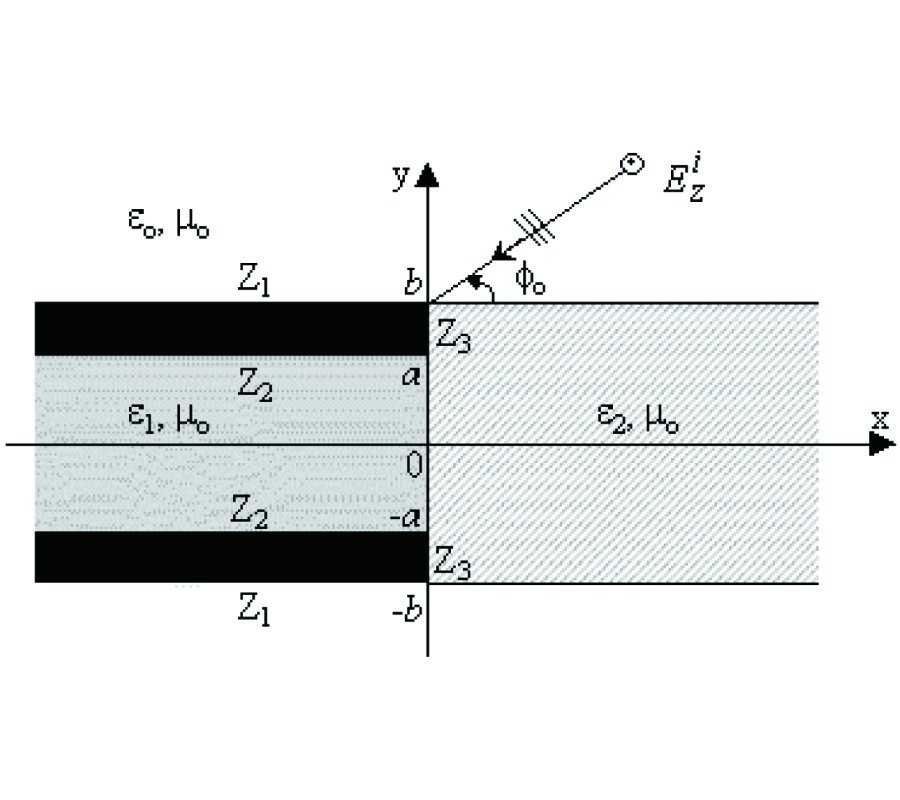Plane Wave Diffraction by Dielectric Loaded Thick-Walled Parallel-Plate Impedance Waveguide

The high frequency diffraction of Ez-polarized plane waves by a dielectric loaded thick-walled parallel-plate impedance waveguide is investigated rigorously by using Fourier transform technique in conjunction with the mode-matching method. Relying upon the image bisection principle, the original problem is splitted up into two simpler ones and each individual boundary-value problem is formulated with this mixed method which gives rise to a scalar Wiener-Hopf equation of the second kind. The solution of each Wiener-Hopf equation contains infinitely many constants satisfying an infinite system of linear algebraic equations. A numeric solution of this system is obtained for various values of the dielectric constant, plate impedances plate thickness, and the distance between the plates through which the effect of these parameters on the diffraction phenomenon is studied.

PIER Vol. 44, 131-142, 2004. doi:10.2528/PIER03062001

###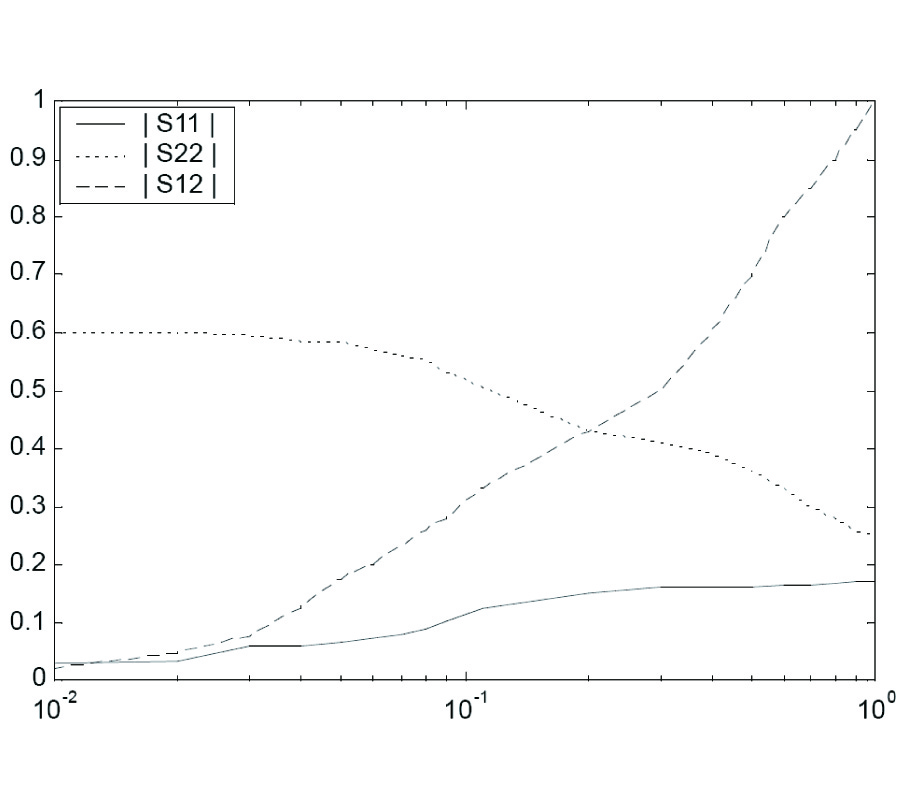Numerical Analysis of Two Dimensional Tapered Dielectric Waveguide

Asok De and Girish Attimarad

A simple method is presented to obtain the scattering parameters of the two dimensional tapered dielectric waveguide, by discrete approximation to tapering, consisting of series of steps. The two dimensional step discontinuity of the junction of two different dielectric rectangular waveguides has been solved using integral equation arising from the field matching of the discrete modes and the continuous spectrum. Accurate numerical solution has been obtained using Ritz-Galerkin variational approach with appropriate sets of expanding functions. The results in the form of scattering parameters for varying tapered length have been depicted graphically. Computed results from generalized integral expressions are found to be in excellent agreement with results obtained in two-dimensional case. With this method it is possible to design the structure to enlarge the cross section of a mode in a slow and controlled manner.

PIER Vol. 44, 103-129, 2004. doi:10.2528/PIER03032002

###Scattering of X-Waves from a Circular Disk Using a Time Domain Incremental Theory of Diffraction

The diffraction and scattering of a first-order ultrawideband TE X-wave by a perfectly conducting circular disk is investigated using an augmented time-domain incremental theory of diffraction. The analysis relies on a pulsed plane wave representation of the incident X-wave. The diffraction and scattering of each constituent pulsed plane wave component is calculated at the observation point. A subsequent azimuthal angular superposition yields the diffracted and scattered field due to the incident X-wave pulse. Making use of the localization and symmetry properties of the incident TE X-wave, a novel four-sensor correlated detection scheme is introduced which is particularly effective in detecting the edges of the scattering disk and has an exceptional resolving power.

PIER Vol. 44, 81-101, 2004. doi:10.2528/PIER03032001

###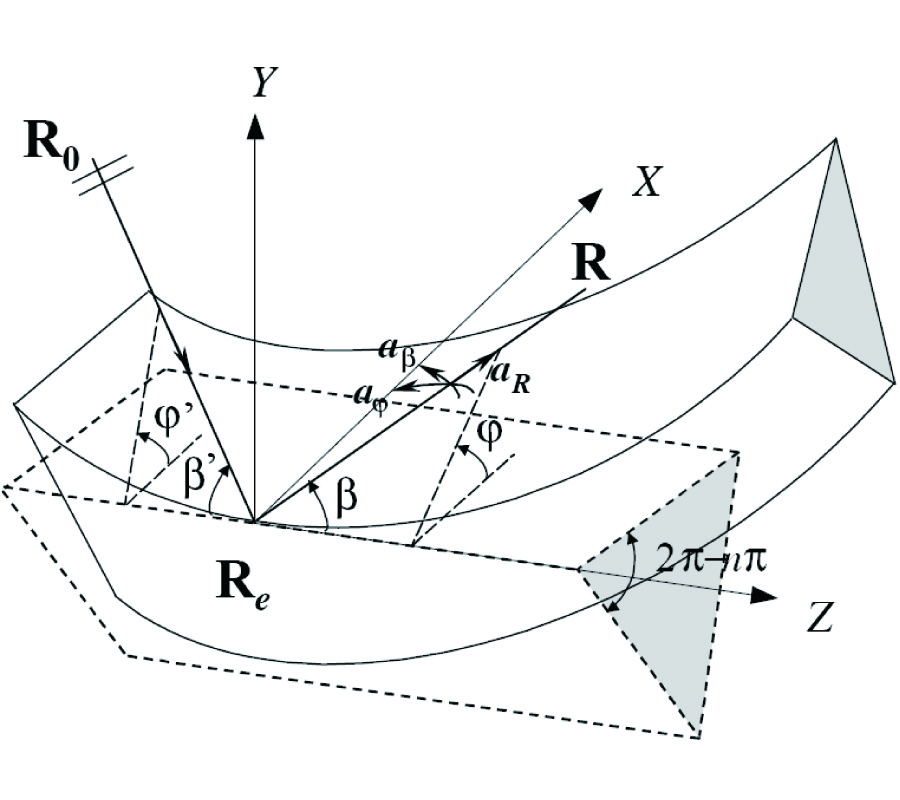A Time Domain Incremental Theory of Diffraction: Scattering of Electromagnetic Pulsed Plane Waves

A new formulation of a time domain incremental theory is introduced. This approach is applied to the scattering of a pulsed plane wave incident on a circular disk. It is shown that the scattered field is free from singularities at caustics and exhibits a notable wave structure outside Keller's cone.

PIER Vol. 44, 57-79, 2004. doi:10.2528/PIER03030601

###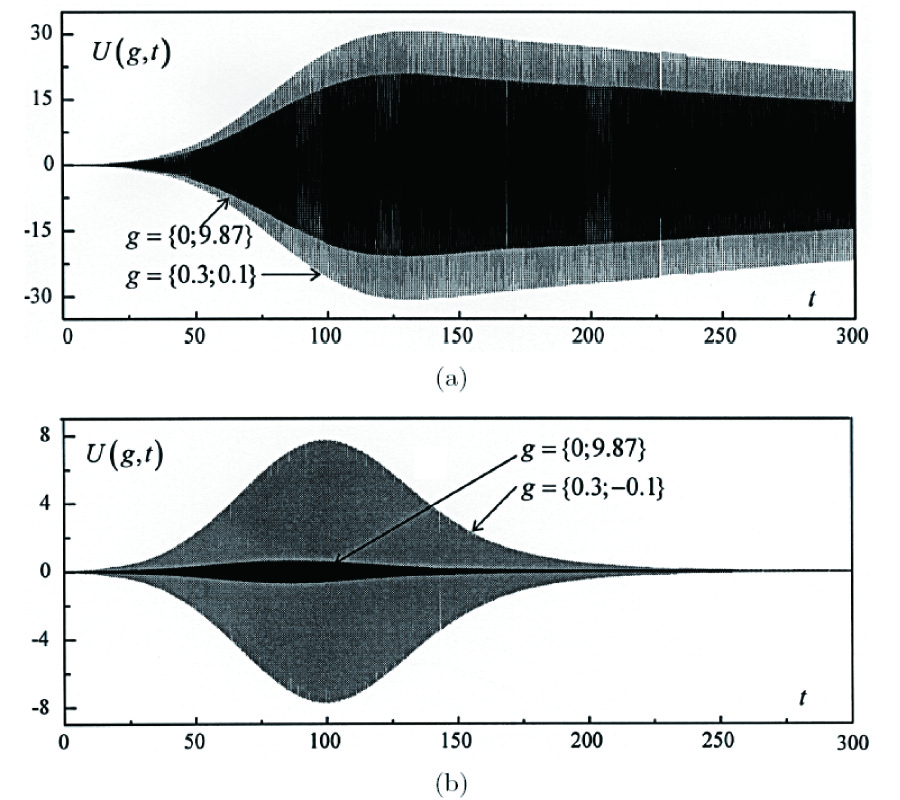Time-Domain and Frequency-Domain Methods Combined in the Study of Open Resonance Structures of Complex Geometry

The paper discusses the methodological questions arising in the study of open electrodynamic structures of resonance quasi optics via time-domain technique. As demonstrated, all of the interesting physical characteristics inherent in these objects (including the objects with various frequency-selective elements) can be obtained through the numerical solution of the relevant model initial bowldaryvalue probhxns. For the first time, a finite difference method equipped with the exact local 'absorbing' conditions on artificial boundaries has been applied for the solution of this kind of open problems. The results of the computational experiments performed have verified the possibility of the efficient selection of oscillations in dispersive open resonators with diffraction gratings, among them the resonators with gratings operating in the quasitotal nonspecular reflection mode.

PIER Vol. 44, 39-55, 2004. doi:10.2528/PIER03022203

###Analysis of Aperture Antennas Above Lossy Half-Space

This paper studies the radiation properties of aperture antennas above imperfect ground using Discrete Complex Image Method (DCIM). The present method is simple and has high accuracy. In this approach, based on linear approximating a function to an exponential series, equivalent complex images have been obtained. Number, intensity and location of images are obtained using Generalized Pencil Of Function (GPOF) technique. We assume current distribution over the aperture be combination of electric and magnetic currents in vertical and horizontal direction. The obtained results are comparable with analytical computation in limited cases. In spite of Sommerfeld integral based methods, this method is simple with lower computational time.

PIER Vol. 44, 1-38, 2004. doi:10.2528/PIER03050602

###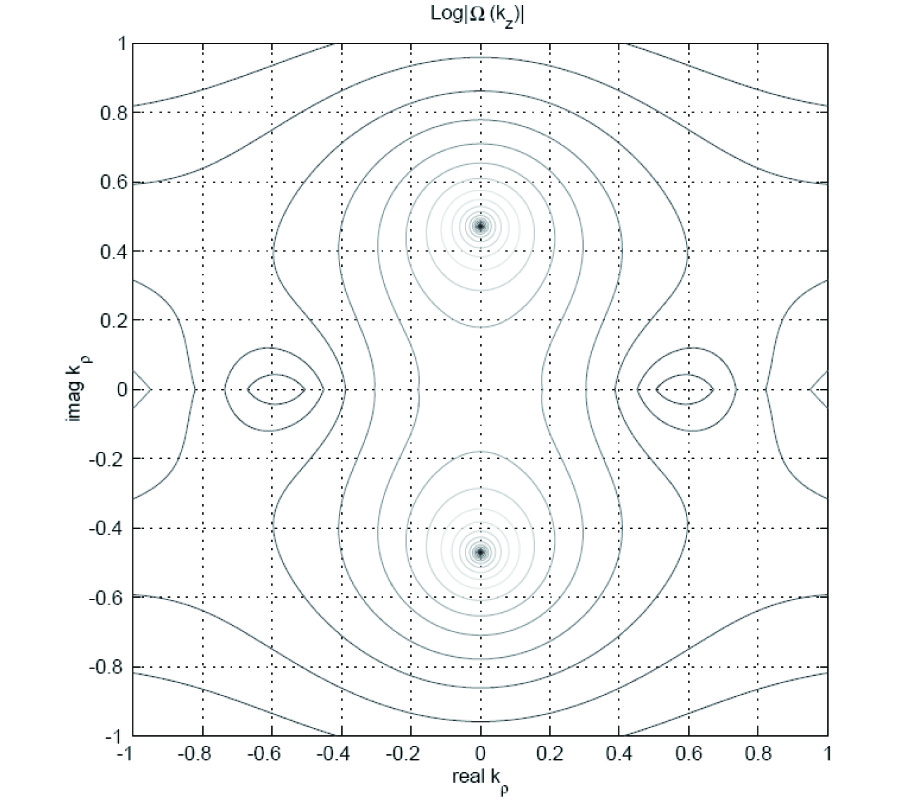A Fast Multipole Method for Embedded Structure in a Stratified Medium

An efficient, static fast multipole method (FMM) based algorithm is presented in this paper for the evaluation of the parasitic capacitance of 3-D microstrip signal lines embedded in stratified dielectric media. The effect of dielectric interfaces on the capacitance matrix is included in the stage of FMM when outgoing multipole expansions are used to form local multipole expansions. The algorithm retains O(N) computational and memory complexity of the free-space FMM, where N is the number of conductor patches.